## 3.2 Kinematic Formulas

To provide the equations of motion, we use the Ricci identities for the vector field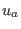: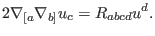(44)

We substitute the vector fieldfrom the kinematic quantities, using the Einstein equation, and separating out the orthogonally projected part into trace, symmetric traceless, and skew symmetric parts. We obtain constraints and propagations for the kinematic quantities as follows:(45)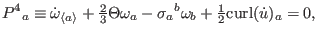(46)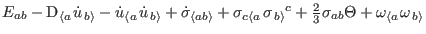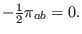(47)

Equation (45), called the Raychaudhuri propagation formula, is the basic equation of gravitational attraction. In Eq. (46), the evolution of vorticity is conserved by the rotation of acceleration. Equation (47) shows that the gravitoelectric field is propagated in shear, vorticity, acceleration, and anisotropic stress.

The Ricci identities also provide a set of constraints: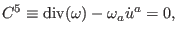(48)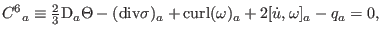(49)(50)

Equation (48) presents the divergence of vorticity. Equation (49) links the divergence of shear to the rotation of vorticity. Equation (50) characterizes the gravitomagnetic field as the distortion of vorticity and the rotation of shear.

Ashkbiz Danehkar
2018-03-26